# BODMAS And Simplification Of Brackets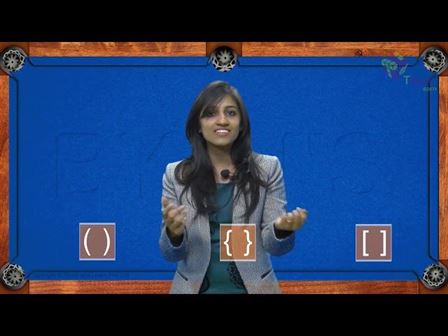An arithmetic expression involving multiple operations is not as simple as operations involving two numbers. An operation on two numbers is easy but how to solve an expression with brackets and multiple operations and how to simplify a bracket? Let’s recollect BODMAS rule and learn about simplification of brackets.

## BODMAS RULE

BODMAS is an acronym and it stands for Bracket, Of, Division, Multiplication, Addition and Subtraction. In certain regions, PEDMAS (Parentheses, Exponents, Division, Multiplication, Addition and Subtraction) is the synonym of BODMAS.

It explains the order of operations to solve an expression. According to Bodmas rule, if an expression contains brackets ((), {}, []) we have to first solve or simplify the bracket followed by of (powers and roots etc.), then division, multiplication, addition and subtraction from left to right. Solving the problem in the wrong order will result in a wrong answer.

Note: The “of” in the BODMAS full form is also called “Order”, which refers to the numbers which involve powers, square roots, etc. Check the examples below to have a better understanding of using the BODMAS rule.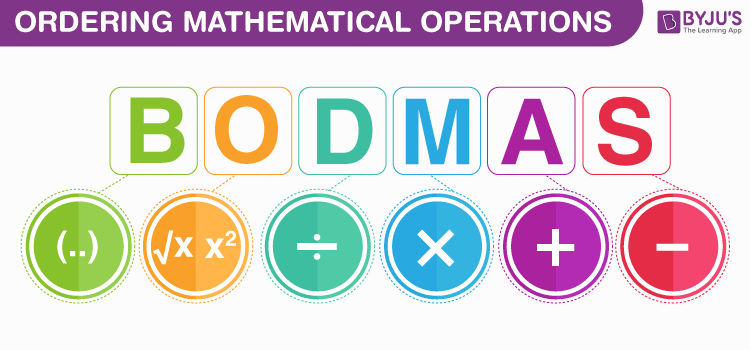Example- Solve $\left ( \frac{1}{2} + \frac{1}{4} \right ) of 16$

Solution-  $\left ( \frac{1}{2} + \frac{1}{4} \right ) of 16$

Step 1: Solving the fraction inside the bracket first-

$\frac{1}{2} + \frac{1}{4} = \frac{3}{4}$

Step 2: Now the expression will be $\frac{3}{4} of 16$ $\frac{3}{4} \times 16$ $= 12$

### SIMPLIFICATION OF BRACKETS

Simplification of brackets in an expression means expansion of brackets. We can remove brackets from an expression by expanding them by multiplication. We use distributive law of multiplication over addition or subtraction. Generally, it can be written as: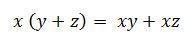Notes: The order of brackets to be simplified is (), {}, [].

Example 2: Expand and simplify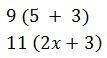Solution:

1. The terms inside the bracket are like terms. We can solve this by two methods.

Method 1:  Add the terms inside the bracket and multiply the number outside bracket with the sum.Method 2: Multiply the number outside the bracket with each term inside the bracket and add the products.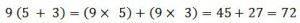1. Here the terms are unlike. This can be simplified by multiplying the number outside the bracket with the terms inside the bracket and add the products.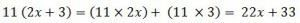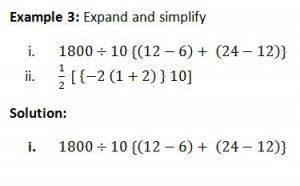Step 1: Simplify the terms inside {}.

Step 2: Simplify {} and operate with terms outside the bracket.

$1800 \div 10{(12-6)+ (24-12)}$

$1800 \div 10 \left \{ 6 + 12 \right \}$

$\Rightarrow 180 \left \{ 18 \right \}$

$= 3240$(ii) $\frac{1}{2}\left [ \left \{ -2(1+2) \right \} 10\right ]$<

Step 1: Simplify the terms inside () followed by {}, then [].

Step 2: Operate terms with the terms outside the bracket.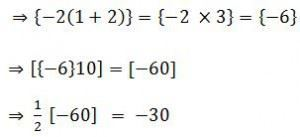Condition Rule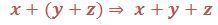Open the bracket and add the terms.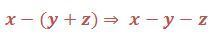Open the bracket and multiply the negative sign with each term inside the bracket. (All positive terms will be negative and vice-versa)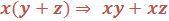Multiply term outside with each term inside the bracket

To solve more word problems on arithmetical operations, download BYJU’S – The Learning App and watch interactive videos.

1. Kenny Matz

8/2(2+2)

1. balaji

2. pardeep

8/2(2+2)
8/2(4)
4*4=16 Ans.

2. Manish

Can anyone help:

18/3(5-4+1)

1. balaji

3. Srinivas

for question 2:
18/3(5-4+1)
If the expression is 18/3(5-4+1), then solution will be
= 18/3*(2)
=18/6 =3

If the expression is (18/3). (5-4+1), the solution will be
= (6)* (2) =12

4. Ghz

626352/6243(72+2651)

1. srinivas

For this question – 626352/6243(72+2651), explanation below
= (626352/6243) * 2723
= (208784/2081) * 2723
Since, a * b/c = (a * b)/c
= (208784/2081) * 2723 = 568518832 / 2081
= 273195.01778